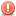# Thread: How to calculate the time difference

1. ##Does anybody know how to calculate the time difference between (for example: 01:00 PM and 02: AM)?
I've set 2 variables:

StartTimeValue = Format(MyStartTime, "hh")
EndTimeValue = Format(MyEndTime, "hh")

'StartTimeValue will return 13 when 01:00 PM is set.
'EndTimeValue will return 02 when 02:00 AM is set.

Now how can I get the time difference? Is this format
I'm using ok, or should I use "hh:mm" for the return
value?

thx, sub-zero

Visual Studio 6.0 Enterprise Edition2. Try this.
Code:
```'When bMethod = False, the operation is subtraction.
'When bMethod = True, the operation is addition
Function TimeDiff(dTime1 As Date, dTime2 As Date, bMethod As Boolean) As Date
dtTime1 = Format("3:00:00 AM", "hh:mm:ss")
dtTime2 = Format("1:00:00 AM", "hh:mm:ss")
If bMethod = True Then TimeDiff = CDate(dTime1 + dTime2)
If bMethod = False Then TimeDiff = CDate(dTime1 - dTime2)
End Function```
Usage:
Code:
```'Subtracts 2:00 from 3:00
Print TimeDiff("3:00", "2:00", False)```3. This will return the amount of hours between the two time points.

Code:
```StartTimeValue = Format("1:00:00 AM", "h:mm:ss AMPM")
EndTimeValue = Format("2:00:00 AM", "h:mm:ss AMPM")
RetVal = DateDiff("h", StartTimeValue, EndTimeValue)
MsgBox RetVal```4. ##I allready tried this in similary methods but the
problem is - it seams that the application cannot
detect the difference between AM and PM.

When I use one of this functions, or any other
it would return the following:

Definitions:
------------
StartTimeValue = Format(MyTime, "hh")
EndTimeValue = Format(MyTime, "hh")

'MyTime for StartTimeValue could be 01:00 PM and would
return 13.
'MyTime for EndTimeValue could be 02:00 AM and would
return 02.

When I try to get the difference, it returns a difference
of 11 hours for 01:00 PM and 02:00 AM.
That is correct when you say, you start at 02:00 AM and
count the hours until 01:00 PM.

What I want to do is to count the hours from 01:00 PM to
02:00 AM next day. Then it would result a of 13 hours
difference.

Any other suggestions?

thx, sub-zero5. ##Sorry, but it doesn't. When I try to get the difference
between 01:00 PM (current day) and 02:00 AM (next day)
with this code it returns 1.
Then my brain says NO - there can't be 1 hour difference.
That only would be the difference between 1 and 2.

thx, sub-zero6. ##OK guys! - I just got it. Don't need that code.
When I calculate 24 - StartTimeValue + EndTimeValue
then it returns the difference.
Sometimes it's as easy as you would think.

thx, sub-zero7. ## Re: How to calculate the time differenceOriginally Posted by vbzeroOK guys! - I just got it. Don't need that code.
When I calculate 24 - StartTimeValue + EndTimeValue
then it returns the difference.
Sometimes it's as easy as you would think.

thx, sub-zero
I'm not sure that your previous code will work quit well

but try this :
============
'Here you go :

Dim ADate, A1Date, BDay, B1Day, CMonth, C1Month, DYear, D1Year

'If you want to calculate time diff in the same day, just delete the "+ 1"

B1Day = Day(A1Date)
C1Month = Month(A1Date)
D1Year = Year(A1Date)

Dim MystrtTime, MyendTime, strtDATEnTIME, endDATEnTIME, RetVal, GetMinDiff, GetHours, GetMinutes, FinalDiff

MystrtTime = Format("06:10:00 PM", "hh:mm:ss AM/PM")
MyendTime = Format("06:50:00 AM", "hh:mm:ss AM/PM")

endDATEnTIME = Format(B1Day & "-" & C1Month & "-" & D1Year & " " & MyendTime, "dd-mm-yyyy hh:mm:ss")
strtDATEnTIME = Format(BDay & "-" & CMonth & "-" & DYear & " " & MystrtTime, "dd-mm-yyyy hh:mm:ss")

' Get Hours
GetHours = Int(((DateDiff("s", strtDATEnTIME, endDATEnTIME) / 60) / 60))

'Get Minutes
GetMinDiff = Int(((((DateDiff("s", strtDATEnTIME, endDATEnTIME) / 60) / 60)) - Int(((DateDiff("s", strtDATEnTIME, endDATEnTIME) / 60) / 60))) * 60)

If GetMinDiff >= 60 Then
GetMinutes = GetMinDiff - 60
GetHours = GetHours + 1
Else
GetMinutes = GetMinDiff
End If

FinalDiff = GetHours & ":" & GetMinutes

RetVal = Format(FinalDiff, "hh:mm:ss")

MsgBox RetVal

' Done

BR,
Mous8. ## Re: How to calculate the time difference

Welcome to the forums MousI don't think he still needs help with this (14 years later). See the main page of this Forum, there you'll find many active threads.9. ## Re: How to calculate the time difference

AND, you have some issues with the way you are declaring variables.....:-)10. ## Re: How to calculate the time difference

Thanks for your repliesI know its too old , but almost every one use your forum as a reference so I wanted to complete the thread.

dear sam ,
would you please fix my code issues , so I could learn from you , and other Google searchers will find a good complete articleThank you again.11. ## Re: How to calculate the time difference

Well, I won't go through all that code, but I would suggest you read this about declaring variables:
(In Visual Basic 6.0, you can declare variables of different types in the same statement, but you must specify the data type of each variable or it defaults to Variant.)

http://msdn.microsoft.com/en-us/libr...(v=vs.90).aspx12. ## Re: How to calculate the time difference

We have a policy not to resurrect threads that are older than one year. Thread closed.####Posting Permissions

• You may not post new threads
• You may not post replies
• You may not post attachments
• You may not edit your posts
•

Featured